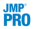Publication date: 07/30/2020

# Exact TestThe following Exact tests are available in the Contingency platform:

Fisher’s Exact Test

Performs Fisher’s Exact test for an r x c table. This is a test for association between two variables. Fisher’s exact test assumes that the row and column totals are fixed, and uses the hypergeometric distribution to compute probabilities.

This test does not depend on any large-sample distribution assumptions. This means it is appropriate for situations where the Likelihood Ratio and Pearson tests become less reliable, like for small sample sizes or sparse tables.

The report includes the following information:

Table Probability (P)

Gives the probability for the observed table. This is not the p-value for the test.

Two-sided Prob P

Gives the p-value for the two-sided test.

For 2x2 tables, the Fisher’s Exact test is automatically performed, unless one row or column contains all zeros (in this case, the test cannot be calculated). See Tests.

Exact Cochran Armitage Trend Test

Performs the exact version of the Cochran Armitage Trend Test. This test is available only when one of the variables has two levels. For more information about the trend test, see Cochran Armitage Trend Test.

Exact Agreement Statistic

Performs an exact test for testing agreement between variables. This is an exact test for the Kappa statistic. This is available only when the two variables have the same levels. For more information about agreement testing, see Agreement Statistic.